# Pie Charts Solved Examples

DIRECTIONS for questions 1-5: Go through the following pie chart and answer the questions given below that.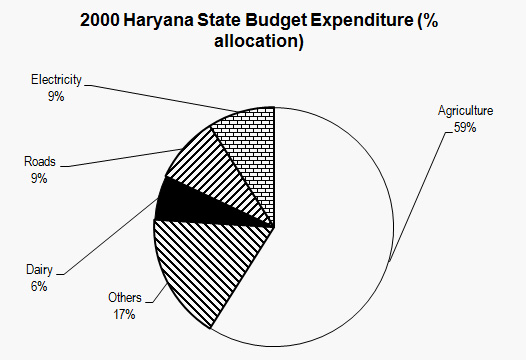Example 1: Approximately how many degrees should be there in the central angle of the sector for agriculture expenditure?
1. 220
2. 213
3. 210
4. 208

Sol: In a pie chart, 100% is spread over 360°. Therefore 1 % = 3.6°. Agriculture expenditure = 59 %. Therefore 3.6 59 = 212.4°. Answer is (2)
Example 2: Approximately what is the ratio of expenditure on agriculture to that on dairy?
1. 8:3
2. 9:2
3. 70:1
4. 10:1

Sol: Over here, one common mistake is that students calculate the actual values of agriculture and dairy. Since budget expenditure is proportional to % of area covered, ratio of agriculture to dairy expenditure would be ratio of corresponding % allocations. Therefore, Agriculture/Dairy = 59/6 = 10/1. Answer is (4).
Example 3: In Haryana, in 2000, a total expenditure of Rs. 120mn was incurred. Approximately how many million did the Haryana government spend on roads?
1. 15.4
2. 20
3. 10.8
4. 12

Sol: Total expenditure = 100% = Rs. 120 mn. Expenditure on roads = 9% = 9/100 × 120 = Rs. 10.8mn. Hence the answer is 3rdoption.
Example 4: If Rs. 9mn were spent in 2000 on Dairy, what would have been the total expenses in that year in million?
1. 150
2. 140
3. 160
4. 130

Sol : 9mn were spent on dairy. This amount represents 6% of total expenditure in the year 2000.
6 = (Dairy expenditure/Total expenditure ) × 100
6 = (9/Total expenditure ) × 100
Total Exp = 100 × (9/6) = Rs. 150mn. Hence, answer is option 1.
Example 5: In 1999, Haryana spent 11% of all its expenses on Roads. “Did Haryana spend more on Roads expenditure in 1999 than in 2000?” To answer the question, we
1. Do not require any additional data.
2. Require to know the total amount spent in each year.
3. Require the exact %age break-up of the various items of expenses in 1999.
4. Require the expenditure on dairy in both the years.
Sol: % gives us just a proportional indication of total quantity.
Unless total expenditure is known, % of that is meaningless.
Hence, to compare expenditure on roads in 1999 and 2000, the total amount spent must be known.
DIRECTIONS for questions 6-10: Refer to the pie charts given below and answer the questions that follow.
YEAR 1995, REGIONAL MARKET SHARES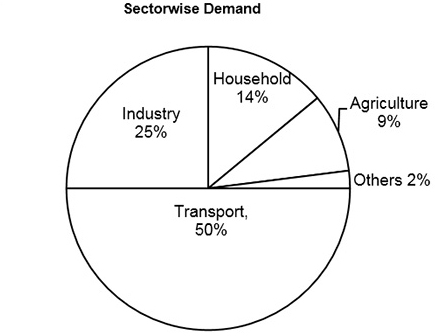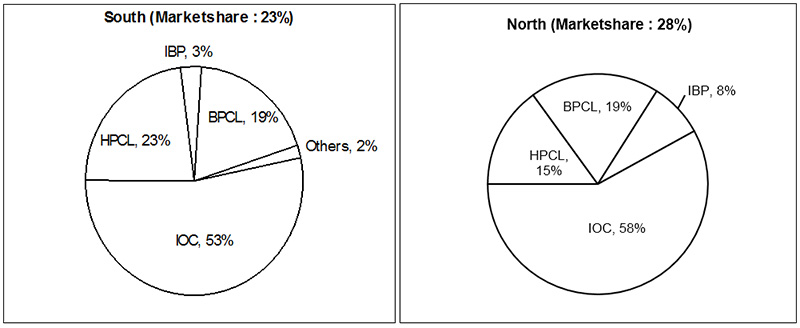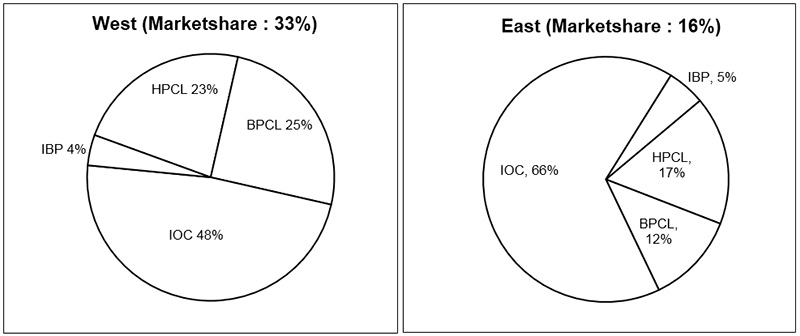The pie charts above give the sales wise distribution (Market shares) of the major oil producing giants in India. The Total sales for these major oil giants (all over India) in 1995 were $600 bn (where$ 1bn = Rs. 3,300 crores). The total demand for oil in 1995 was 100,000 crore kgs (all India).
Example 6: It can be inferred from the graphs that in 1995
1. IOC is the largest oil company in India.
2. HPCL is the smallest oil company in India
3. The greatest consumption of oil is by the ‘agriculture’ sector.
4. None of the above.
Sol: From the pie charts, we can see that IOC had more than 50% share in all regions. So, we can safely say that IOC is the largest company.
Example 7: If total sales (in kg), all over India, by the given oil giants in 1995 is 80% of the total demand, the price of oil (on an average) in 1995 must have been
1. Rs. 18.2 / kg
2. Rs. 13.6 / kg
3. Rs. 15.84 / kg
4. None of these
Sol: Total sales = $600 bn. Total demand = 100000 crore kgs. Total sales = 80% of 100000 = 80000 crore kgs. Price of oil = Rs. (600 × 3300 crore) / 80000 crore kgs = Rs. 24.75 /kg. So answer is 4th option Example 8: The total all India sales of IOC were approximately 1.$ 300 bn
2. $310 bn 3.$ 331.2 bn
4. $340.1 bn Sol: Directly calculate from all the four graphs and add. Total Sales of IOC=$ 600 (0.53×0.23+0.28×0.58+0.48 ×0.33+ 0.16 ×0.66) bn
= 600 (0.1219+0.1624+0.1584+0.1056)
=$328.9 bn =$329 bn(Approx.)
Example 9: The sales of HPCL in West exceeded the sales of BPCL in North by
1. Rs. 3,397crores
2. Rs. 44946 crores
3. Rs. 5,479 crores
4. $4.3 bn Sol: Sales of HPCL in West =$ 600bn × 0.33 × 0.23
= $45.54 bn. Sales of BPCL in North =$ 600bn × 0.28 × 0.19
= $31.92 bn. Difference =$ 13.62bn = Rs. 13.62 × 3300 crore
= Rs. 44946crore. So answer is 2nd option.
Example 10: The total all India sales of all the given companies other than IOC in 1996 was
1. $268.8 bn 2.$ 372.9 bn
3. \$ 280 bn
4. None of these
Sol: For 1996 we don’t have any data. So answer is 4th option.
DIRECTIONS for questions 11-14: Analyze the following pie chart and answer the questions given below. Given is the distribution of the monthly family budget of a person X. The total earnings of person X are Rs. 3,600 per month basic, plus 10% as transport and meals allowance on the monthly salary.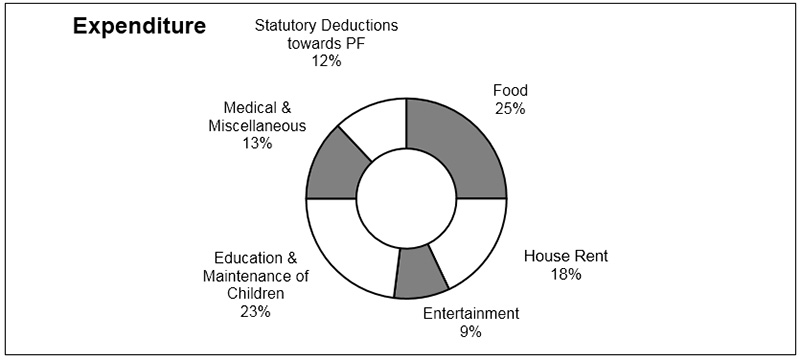Example 11: Calculate the amount of expenditure on Education and Maintenance per month,if a person X pays 23% of its total earnings as Education and Maintenance of children?
1. Rs. 910.8
2. Rs. 900
3. Rs. 920
4. Rs. 915
Sol: Total Emoluments = 3600+ 10% of 3600 = Rs. 3960
23% of 3960 = Rs. 910.8
Example 12: What is the medical and miscellaneous expenditure per annum (in Rs)?
1. 4,854
2. 5,330.16
3. 6177.6
4. 4,485.6
Sol: Total Emoluments = 3600+ 10% of 3600 = Rs. 3960
Medical and Miscellaneous expenditure of total Salary
= 13% of Rs. 3960 = Rs.514.8
Expenditure per annum = Rs. 514.8 × 12 = Rs. 6177.6.
Example 13: What is the approximate earning left of person X (in Rupees) per month after deducting payment of education and maintenance?
1. 3049.2 p.m.
2. 2,591p.m
3. 2,355 p.m.
4. 2,350 p.m
Sol: Total Emoluments = 3600+ 10% of 3600 = Rs. 3960
Education and Maintenance of children =23% of 3960 = Rs. 910.8
Take Home Pay Packet = Rs. 3960 - 910.8
= Rs. 3049.2
Example 14: What is the total amount per month the family spends on house rent and statutory deductions towards PPF expenses?
1. Rs. 1,100
2. Rs. 1,259
3. Rs. 1,188
4. Rs. 1,140
Sol: Expenditure on house = Rs. 712.8
Expenditure on statutory deductions towards PPF expenses=475.2
⇒ Total on both = 712.8 + 475.2 = 1188